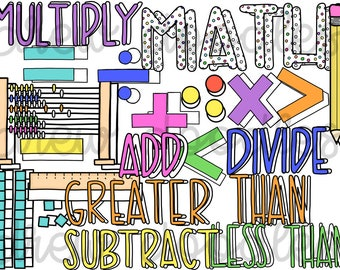### Third Grade Math3rd Grade Go Math Interactive Lessons
3rd Grade Go Math Animated Math Models

Third-grade students will be...

*Developing understanding of multiplication and division
and strategies for multiplication and division within 100.

*Developing understanding of fractions,
especially unit fractions.

*Developing understanding of the structure of
rectangular arrays and area.

*Describing and analyzing two-dimensional shapes.